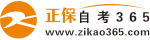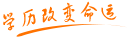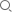#24小时客服：4008135555/010-82335555# 自考“C语言程序设计”模拟试题十四

2007年05月29日    来源：   字体：   打印

一、选择题（1—14每题1分，15-18每题1.5分，共20分）

1.可选作用户标识符的一组标识符是（     ）

A void    B c5_b8     C For     D 3a

Define    _53      -ab      DO

WORD      IF       Case     int

2.在C语言中，非法的八进制是：（     ）

A.018    B.016    C.017    D.0257

3.在TC中，基本int a类型变量所占的字节数是（     ）

A.1    B.2    C.4    D.8

4.设x，y，z，k都是int型变量，则执行表达式：x=（y=4，z=16，k=32）后，x的值为（   ）

A.4    B.16    C.32    D.52

5.设int型变量a为5，使b不为2的表达式是（     ）

A.b=a/2   B.b=6-（-a）    C.b=a%2    D.b=a>3？2：1

6.一个C程序的执行是从（     ）

A.main（）函数开始，直到main（）函数结束。

B.第一个函数开始，更后一个函数结束。

C.第一个语句开始，更后一个语句结束。

D.main（）函数开始，直到更后一个函数。

7.C语言中用于结构化程序设计的三种基本结构是（     ）

A.if、switch、break

B.if   while   for

C.while   do- while   for

D.顺序结构、选择结构、循环结构

8.以下叙述中不正确的是（     ）

A.在不同的函数中可以使用相同名字的变量。

B.函数中的形式参数是局部变量。

C.在一个函数内的符合语句中定义变量在本函数范围内有效。

D.在一个函数内定义的变量只在本函数范围内有效。

9.若k为int 类型，且k的值为3，执行语句k+=k-=k*k后，k的值为（     ）

A.-3    B.6    C.-9    D.-12

10.有以下程序

main（）

{int x=3，y=3，z=3；

printf（“%d   %d\n”（++x，y++），++z）；} 输出结果（     ）

A.3  3    B.3  4

C.4  2    D.4  3

11.若有定义和语句：int a=21，b=021； printf（“%x，%d \n”，a，b）；输出结果（ ）

A.17，15     B.16，18

C.17，19     D.15，17

12.已有定义语句：int x=3，y=4，z=5；则值为0的表达式是（     ）

A.x>y++           B.x<=++y

C.x！=y+z>y-z     D.y%z>=y-z

13.能正确表达逻辑关系“a≥10或a≤0”的C语言表达式是（     ）

A.a>=10 or a<=0    B.a>=10||a<=0

C.a>=10&&a<=0     D.a>=10|a<=0

14.n为整型常量，且n=2；while（n——）；printf（“%d”，n）；执行后的结果是：（     ）

A.2     B.1     C.-1    D.0

15.若有以下定义和赋值double  *q，a=5.5； int  *p，i=1； double  *q，a=5.5；int *p，i=1； p=&i； q=&a； 以下对赋值语句叙述错误的是（    ）。

A）*p=*q     变i中的值。

B）p=oxffd0；将改变p的值，使p指向地址为ffd0的存储单元。

C）*q=*p；等同于a=i；

D）*p=*q；是取q所指变量的值放在p所指的存储单元

16.若有以下定义语句double a，*p=a；   int i=5； 对数组元素错误的引用是（    ）

A）*a   B） *a   C）*（p+i）   D）p

17.以下选项中不能使指针正确指向字符串的是（    ）

A）char  *ch；*ch=“hello”

B）char  *ch=“hello”

C）char  *ch=“hello”；ch=“bye”

D）char  *ch“；ch=”hello“

18.若有以下说明和定义语句：union  uti {int n；double g；char ch；}

struct  srt{float xy；union  uti  uv；}aa；则变量aa所占内存的字节数是（    ）

A） 9     B） 8    C） 13    D） 17

二、填空题（每空1分，共12分）

1.C语言源程序文件的扩展名是 （1） ，经过编译后，生成文件的扩展名是 （2） ，经过连接后，生成文件的扩展名是 （3）.

2.把a，b定义成长整型变量的定义语句是 （4）

3.设x和y均为整型变量，且x=3，y=2，则1.0*x/y表达式的值为 （5）

4.已有定义：float x=5.5；则表达式：x=（int）x+2的值为 （6）

5.已有定义：int x=0，y=0；则表达式：（x+=2，y=x+3/2，y+5）后，变量x的值为 （7），变量y的值为 （8），表达式的值为 （9） .

6.执行以下for（i=1；i++<=5）语句后，变量i的值为（10）.

7.数组是表示类型相同的数据，而结构体则是若干 （11）数据项的集合。

8.C语言中文件是指     （12）       .

三、程序补充题（每空3分，共24分）

1.从键盘上输入若干个学生成绩，统计并输出更高成绩和更低成绩，当输入负数时结束输入。

main（）

{ float s，gmax，gmin；

scanf（“%f，”&s）；

gmax=s；gmin=s；

while  （1）

{if（s>gmax）gmax=s；

if  （2）     gmin=s；

scanf（“%f”，&s）；}

printf（“gmax=%f\ngmin=%f\n”gmax，gmin）；}

2.求任意两个正整数的更大公约数和更小公倍数

#include “stdio.h”

main（）

{int r，m，n，temp，gcd，lcm；

scanf（“   （3）   ”，&m，&n）；

lcm=m*n；

while（m%n！=0）

{r=m%n；（4）；n=r；}

gcd=n；lcm=lcm/n；

printf（“gcd=%d\nlcm=%d\n”，gcd，lcm）；}

3.求y的x次方。

Double fun1（double y，int x）

main（）{int i；

double z=1.0；

for（i=1；i    （5）     ；i++）

z=   （6）     ；

return z；}

4.对输入一个整数进行判断，若是偶数，输出even，否则输出odd，在子函数fun2功能是判定整数是否为偶数，若是偶数，返回1，否则返回0.

int fun2（int x）

{if（x%2==0）  （7）   ；

return  0  ；}

main（）

{int n；

scanf（“%d”，&n）；

if   （8） printf（“even\n”）；

else printf（“odd\n”）；}

四、按格式写出程序运行结果（每题4分共20分）

1.  main（）

{int a=1，c=65，d=97；

printf（“a8=%o，a16=%x\n”，a，a）；

printf（“c10=%d，c8=%o，c16=%x，cc=%c\n”c，c，c，c）；

d++；

printf（“d10=%d，dc=%c\n”d，d）；}

程序运行结果为：

2.有以下程序

void f（int x，int y）

{int t；

if（x<y）{t=x；x=y；y=t；}}

main（）

{int a=4，b=3；c=5；

f（a，b）； f（a，c）； f（b，c）；

printf（“%d，%d，%d”，a，b，c）；}  执行后的结果是：（    ）

3.程序执行的结果是（    ）

main（）

{int i=0，a=2；

if（i==0）printf（“**”）；

else printf（“\$\$”）；

printf（“*”）；}

4.有以下程序

main（）

{int sum=0，n；

scanf（“%d”，&n）；

while（n<=5）

{sum+=n；

n++；}

printf（“sum=%d”，sum）；}

当输入：1   程序运行的结果为（    ）

5.执行以下程序段，输出的结果为（   ）

main（）

{ int a={{3，2，7}，{4，8，6}}；

int *p，m；

p=&a；

m=（*p）*（*（p+2））*（*（p+4））；

printf（“m=%d”，m）；}

五、按题目要求写出可运行程序：（24分）

1.模拟计算器功能，编写程序，根据用户输入的运算符，对两个数进行运算。（用switch语句） （）

2.求3~100之间的全部素数，并统计素数个数。• 购物车
• APP下载扫一扫，立即下载

安卓版本：

苹果版本：

开发者：北京正保自考教育科技有限公司

应用涉及权限：查看权限>>

APP隐私策略：查看政策>>

• 公众号扫一扫，立即关注

• 微信群进群领试题/资料